# if a 35 kg of ice at -10 degrees C is combined with 110 kg of...

if a 35 kg of ice at -10 degrees C is combined with 110 kg of water at 80 degree C, what will be the final equilibrium temperature (in C) of the system?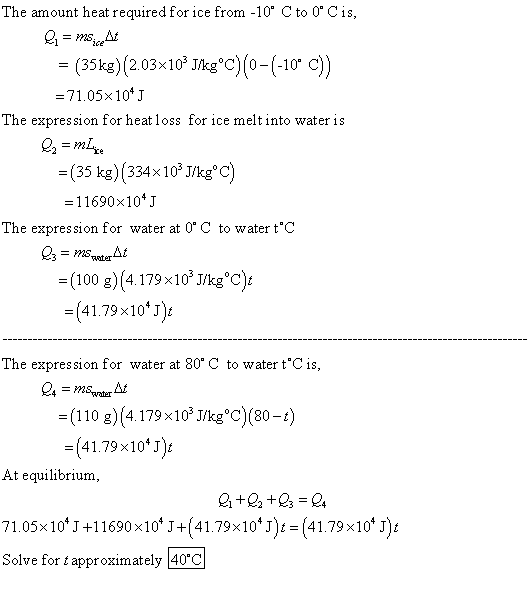2108*(T+10)*35 = 4181*110*(80-T)SOLUTION :

Let tº C be the equilibrium temperature.

Heat absorbed by 35 kg of ice to become water at tº C

= Heat for temperature riseof ice  from - 10º C to 0º C + latent heat to melt ice

+ heat for raising the temperature of melt water from 0º C to tº C .

= 35 * 0.5 * 10 + 35 * 80 + 35 * 1 * t  CHU

(sp. heat of ice = 0.5 and that of water =1.0)

= 2825 + 35 t CHU

Heat given up by 110 kg hot water for fall from 80º C to tº C

= 110 * 1 * (80 - t) CHU

Heat absorbed by ice = Heat given up by hot water.

=> 2825 + 35 t = 8800 - 110t

=> 35t + 110t = 8800 - 2825

=> 145 t = 5975

=> t = 5975/145 = 41.21º C

So, equilibrium temperature will be = 41.21º C (ANSWER).

#### Earn Coin

Coins can be redeemed for fabulous gifts.

Similar Homework Help Questions
• ### Ice with a mass of 0.15 kg at 0.0 degrees Celsius is added to 0.25 kg...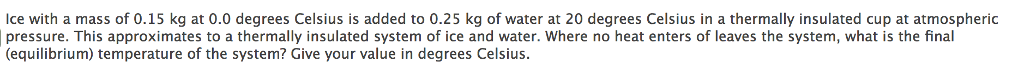Ice with a mass of 0.15 kg at 0.0 degrees Celsius is added to 0.25 kg of water at 20 degrees Celsius in a thermally insulated cup at atmospheric pressure. This approximates to a thermally insulated system of ice and water. Where no heat enters of leaves the system, what is the final (equilibrium) temperature of the system? Give your value in degrees Celsius.

• ### 15 gm of ice at-8 degree C are mixed with 110 gm of water contained in...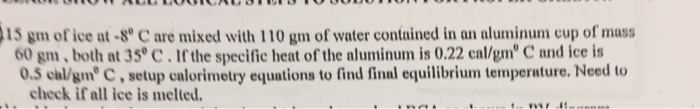15 gm of ice at-8 degree C are mixed with 110 gm of water contained in an aluminum cup of mass 60 gm, both at 35 degree C. If the specific heat of the aluminum is 0.22 cal/gm degree C and ice is 0.5 cal/gm degree C, setup calorimetry equations to find final equilibrium temperature. Need to check if all ice is melted.

• ### A. mass of 3.00 kg of water at 50 degree C has 1.00 kg of ice...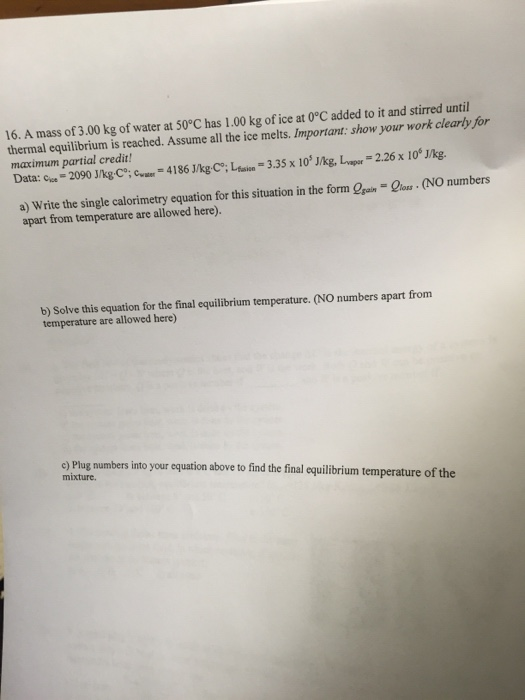A. mass of 3.00 kg of water at 50 degree C has 1.00 kg of ice at 0 degree C added to it and stirred until thermal equilibrium is reached. Assume all the ice melts. Important: show your work clearly for maximum partial credit! Data: c_ice = 2090 J/kg C degree; c_max = 4186 J/kg C degree; L_fusion = 3.35 times 10^5 J/kg. L_vapor = 2.26 times 10^6 J/kg. Write the single calorimetry equation for this situation in the form...

• ### Of ice at -S °C is added to 40 kg of water initially at 20 °C. Assume that the container is well ...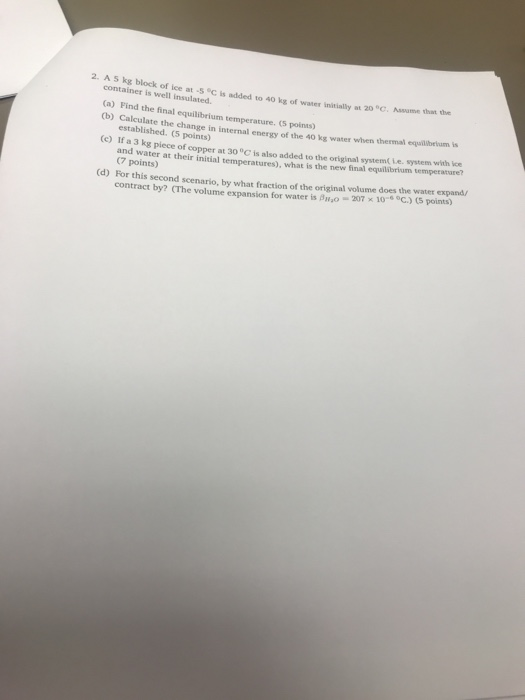of ice at -S °C is added to 40 kg of water initially at 20 °C. Assume that the container is well insulated. (a) Find the final equilibrium temperature. (5 points) 0b) Caleulate the change in internal energy of the 40 kg water when thermal equilberium is established. (5 points) (c) If a 3 kg piece of copper at 30°C is also added to the original system( Le. system with ice d wat an (7 points) er at their initial...

• ### Calculate the final equilibrium temperature when 10.0 grams of steam initially at 100 degree C is...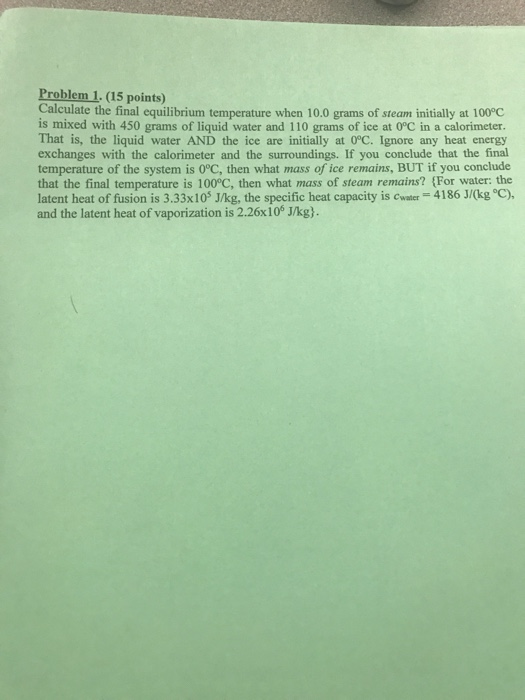Calculate the final equilibrium temperature when 10.0 grams of steam initially at 100 degree C is mixed with 450 grams of liquid water and 110 grams of ice at 0 degree C in a calorimeter. That is, the liquid water AND the ice are initially at 0 degree C. Ignore any heat energy exchanges with the calorimeter and the surroundings. If you conclude that the final temperature of the system is 0 degree C, then what mass of ice remains,...

• ### A 200 g piece of ice at 0 degrees Celsius is place in 500 g of...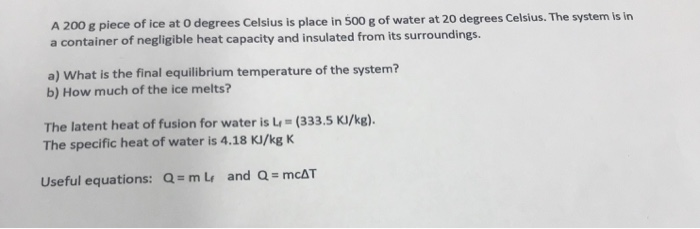A 200 g piece of ice at 0 degrees Celsius is place in 500 g of water at 20 degrees Celsius. The system is in a container of negligible heat capacity and insulated from its surroundings. a) What is the final equilibrium temperature of the system? b) How much of the ice melts? The latent heat of fusion for water is (333.5 KI/kg). The specific heat of water is 4.18 KI/kg K Useful equations: Q = mlf and Q =...

• ### A 0.0725 kg ice cube at −30.0°C is placed in 0.497 kg of 35.0°C water in...

A 0.0725 kg ice cube at −30.0°C is placed in 0.497 kg of 35.0°C water in a very well insulated container, like the kind we used in class. The heat of fusion of water is 3.33 x 105 J/kg, the specific heat of ice is 2090 J/(kg · K), and the specific heat of water is 4190 J/(kg · K). The system comes to equilibrium after all of the ice has melted. What is the final temperature of the system?

• ### A 0.0575 kg ice cube at −30.0°C is placed in 0.617 kg of 35.0°C water in...

A 0.0575 kg ice cube at −30.0°C is placed in 0.617 kg of 35.0°C water in a very well insulated container, like the kind we used in class. The heat of fusion of water is 3.33 x 105 J/kg, the specific heat of ice is 2090 J/(kg · K), and the specific heat of water is 4190 J/(kg · K). The system comes to equilibrium after all of the ice has melted. What is the final temperature of the system?

• ### A 40-g block of ice is cooled to -77degree C and is then added to 620...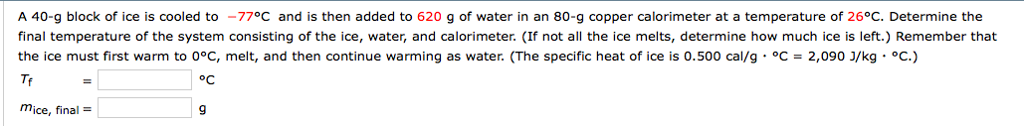A 40-g block of ice is cooled to -77degree C and is then added to 620 g of water in an 80-g copper calorimeter at a temperature of 26degree C. Determine the final temperature of the system consisting of the ice, water, and calorimeter. (If not all the ice melts, determine how much ice is left.) Remember that the ice must first warm to 0degree C, melt, and then continue warming as water. (The specific heat of ice is 0.500...

• ### 2. (5 points) A 35 g ice cube at 0 °C is placed on a 1.5...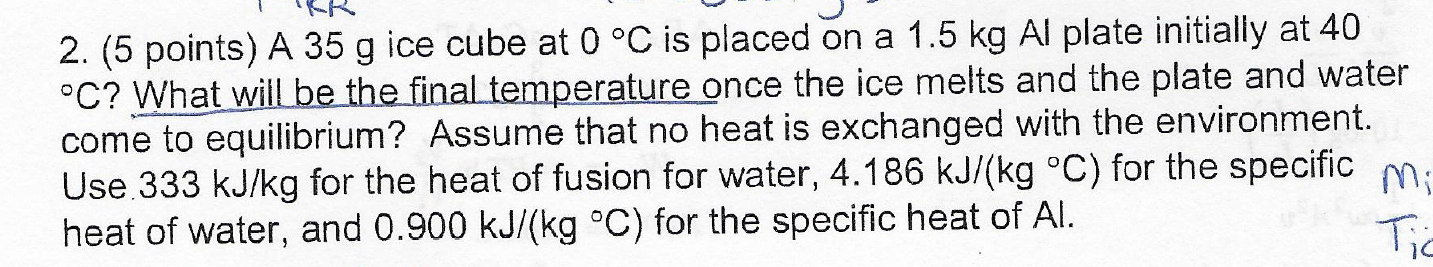2. (5 points) A 35 g ice cube at 0 °C is placed on a 1.5 kg Al plate initially at 40 °C? What will be the final temperature once the ice melts and the plate and water come to equilibrium? Assume that no heat is exchanged with the environment. Use 333 kJ/kg for the heat of fusion for water, 4.186 kJ/(kg °C) for the specific heat of water, and 0.900 kJ/(kg oC) for the specific heat of Al

Free Homework Help App